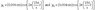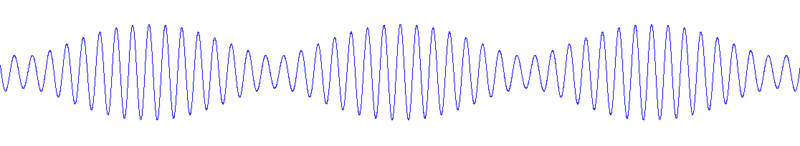# Wave Composition - Different Amplitudes

bobthenormal

## Homework Statement

The superposition of two waves, [see attachment - it doesn't let me link the attachment because I'm new ;P] at the location x = 0 in space results in what kind of wave behavior? [As in, how often does it beat and what frequency is the sound?]

## Homework Equations

Wave equations are given, general form is: Acos(kx-wt).

k = 2pi/lamda; w = 2pi/T = 2pi*f

## The Attempt at a Solution

This is troublesome because I'm not sure how to work with the different amplitudes. I can't think of a way to add them, because of the different amplitudes I can't factor and get a trig identity that is easy to work with.

Essentially I have: A cos(at) + B cos(bt)

But I can rewrite it as: A cos(at) + B cos(at-ct)

= A cos(at) + B ( cos(at)cos(ct) - sin(at)sin(ct) )

= cos(at)(A + B cos(ct)) - B sin(at)sin(ct)

...etc. It just gets uglier.

Any help on this would be greatly appreciated.. although, I'm going to sleep right now, I'll be up in a few hours.

--Bob

#### Attachments

•sjps6e18_mc01_28.jpg
4.5 KB · Views: 523
Last edited:

michalll
The only modification i can think of that helps a bit is:
A cos(at) + B cos(bt) = (A-B) cos(at) + B cos(at) + Bcos(bt) =
(A-B)cos(at) + 2*B cos((at-bt)/2) cos((at+bt)/2)

from where you can see a few things such as the frequency of the sound is roughly (a+b)/2 assuming a is close to b, frequency of the beats is a-b, because of the symmetry and max and min amplitudes of the beats are A+B and A-B respectively.

bobthenormal
Argh, sorry, I don't know how you got the max and min amplitudes being separated by an a-b beat from that equation? Can you explain it a bit more? I kind of see it... but... 2Bcos((a-b)t/2)cos((a+b)t/2) + (A-B)cos(at) seems less friendly than the original equation to me... the 2B with double cos terms are a bit confusing to try to visualize.

I did realize by thinking of the phase difference, that the composition of the beat must have a 6 Hz frequency because of the relation of period and frequency... the beat obviously has a maximum at t=0, so I just have to find the next beat crest. The amount the waves become out of phase increases by increments of (1/150 - 1/156), so they come into phase every (1/150 - 1/156) seconds, which is 6 Hz. - so, is this f_beat = f_1 - f_2 always true regardless of amplitude differences? The book I have doesn't say anything about beating with different amplitude waves, so I'm not sure.

--Bob

michalll
You may look on it as a superposition of a standard 2*B amplitude beat wave and harmonic wave with constant amplitude A-B, if you imagine only the envelopes of these waves it becomes clear that the envelope of the superposed wave is the sum of them.

is this f_beat = f_1 - f_2 always true regardless of amplitude differences?

It is.